## GATE 2008

 Question 1equals
 A 1 B -1 C ∞ D -∞
Engineering-Mathematics       Calculus
Question 1 Explanation:Question 2

If P, Q, R are subsets of the universal set U, then (P∩Q∩R) ∪ (Pc∩Q∩R) ∪ Q∪ Rc is

 A Qc ∪ Rc B P ∪ Qc ∪ Rc C Pc ∪ Qc ∪ Rc D U
Engineering-Mathematics       Sets-And-Relation
Question 2 Explanation:
Given,
(P∩Q∩R)∪(Pc∩Q∩R)∪Qc∪Rc
It can be written as the p.q.r + p'.q.r +q' + r'
=> (p+p').q.r + q' + r'
=> q.r + (q'+r')
=> q.r + q' + r' = 1 i.e., U
 Question 3

The following system of equations

x1 + x2 + 2x3  = 1
x1 + 2x2 + 3x3 = 2
x1 + 4x2 + ax3 = 4

has a unique solution. The only possible value(s) for a is/are

 A 0 B either 0 or 1 C one of 0, 1 or -1 D any real number
Engineering-Mathematics       Linear-Algebra
Question 3 Explanation:
The conjugate matrix [A|B] isWhen a-5 = 0, then rank(A) = rank[A|B]<3,
So infinite number of solutions.
But, it is given that the given system has unique solution i.e., rank(A) = rank[A|B] = 3 will be retain only if a-5 ≠ 0.
 Question 4

In the IEEE floating point representation, the hexadecimal value 0×00000000 corresponds to

 A the normalized value 2 - 127 B the normalized value 2 - 126 C the normalized value + 0 D the special value + 0
Digital-Logic-Design       Number-Systems
Question 4 Explanation:
Value is ±0 if M=0 and E=0.Question 5

In the Karnaugh map shown below, X denotes a don’t care term. What is the minimal form of the function represented by the Karnaugh map?A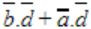BC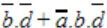DDigital-Logic-Design       K-Map
Question 5 Explanation: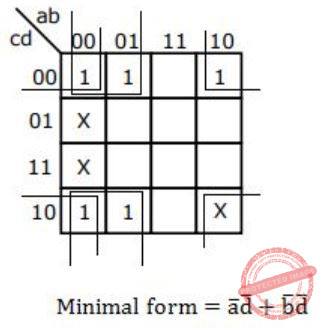Question 6

Let r denote number system radix. The only value(s) of r that satisfy the equationis/are

 A decimal 10 B decimal 11 C decimal 10 and 11 D any value > 2
Digital-Logic-Design       Number-Systems
Question 6 Explanation:
√121r = 11r
(r2 + 2r + 1)1/2 = r + 1
(r + 1)2 * 1/2 = r + 1
r + 1 = r + 1 Any value of r will satisfy the above equation. But the radix should be greater than 2 because the 121 has 2. So r > 2 is correct.
 Question 7

The most efficient algorithm for finding the number of connected components in an undirected graph on n vertices and m edges has time complexity

 A θ(n) B θ(m) C θ(m+n) D θ(mn)
Data-Structures       Graphs
Question 7 Explanation:
To find the number of connected components using either BFS or DFS time complexity is θ(m+n).
Suppose if we are using Adjacency matrix means it takes θ(n2).
 Question 8

Given f1, f3 and f in canonical sum of products form (in decimal) for the circuitf1 = Σm(4,5,6,7,8)
f3 = Σm(1,6,15)
f = Σm(1,6,8,15)

then f2 is

 A Σm(4,6) B Σm(4,8) C Σm(6,8) D Σm(4,6,8)
Data-Structures       Canonical-Normal-Form
Question 8 Explanation:
f = f1* f2 + f3
f1*f2 is intersection of minterms of f1 and f2
f = (f1*f2) + f3 is union of minterms of (f1*f2) and f3
Σm(1,6,8,15) = Σm(4,5,6,7,8) * f2 + Σm(1,6,15)
Options A, B and D have minterm m4 which result in Σm(1,4,6,15), Σm(1,4,6,8, 15) and Σm(1,4,6,8, 15)respectively and they are not equal to f.
Option C : If f2 = Σm(6,8)
RHS: Σm(4,5,6,7,8) * Σm(6,8) + Σm(1,6,15)
= Σm(6,8) + Σm(1,6,15)
= Σm(1,6,8,15)
= f = LHS
 Question 9

Which of the following is true for the language {ap|p is a prime} ?

 A It is not accepted by a Turing Machine B It is regular but not context-free C It is context-free but not regular D It is neither regular nor context-free, but accepted by a Turing machine
Theory-of-Computation       Identify-Class-Language
Question 9 Explanation:
Finding prime number cannot be done by FA or PDA, so it cannot be regular or CFL. This language can be recognized by LBA, hence it can be accepted by Turing Machine.
 Question 10

Which of the following are decidable?

I. Whether the intersection of two regular languages is infinite
II. Whether a given context-free language is regular
III. Whether two push-down automata accept the same language
IV. Whether a given grammar is context-free
 A I and II B I and IV C II and III D II and IV
Theory-of-Computation       Decidability-and-Undecidability
Question 10 Explanation:
The intersection of two regular languages is always a regular language (by closure property of regular language) and testing infiniteness of regular language is decidable. Hence statement I is decidable.
Statement IV is also decidable, we need to check that whether the given grammar satisfies the CFG rule (TYPE 2 grammar productions).
But statements II and III are undecidable, as there doesn’t exist any algorithm to check whether a given context-free language is regular and whether two push-down automata accept the same language.
 Question 11

Which of the following describes a handle (as applicable to LR-parsing) appropriately?

 A It is the position in a sentential form where the next shift or reduce operation will occur. B It is non-terminal whose production will be used for reduction in the next step. C It is a production that may be used for reduction in a future step along with a position in the sentential form where the next shift or reduce operation will occur. D It is the production p that will be used for reduction in the next step along with a position in the sentential form where the right hand side of the production may be found.
Compiler-Design       Parsers
Question 11 Explanation:
A handle is the production p that will be used for reduction in the next step along with a position in the sentential form where the right hand side of the production may be found.
 Question 12

Some code optimizations are carried out on the intermediate code because

 A They enhance the portability of the compiler to other target processors B Program analysis is more accurate on intermediate code than on machine code C The information from dataflow analysis cannot otherwise be used for optimization D The information from the front end cannot otherwise be used for optimization
Compiler-Design       Compilers
Question 12 Explanation:
The code-optimization on intermediate code generation will always enhance the portability of the compiler to target processors. The main reason behind this is, as the intermediate code is independent of the target processor on which the code will be executed, so the compiler is able to optimize the intermediate code more conveniently without bothering the underlying architecture of target processor.
 Question 13

If L and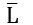are recursively enumerable, then L is

 A regular B context-free C context-sensitive D recursive
Theory-of-Computation       Recursive-Enumerable-Languages
Question 13 Explanation:
If L is recursive enumerable, then it implies that there exist a Turing Machine (lets say M1) which always HALT for the strings which is in L.
Ifare recursively enumerable, then it implies that there exist a Turing Machine (lets say M2) which always HALT for the strings which is NOT in L(as L is complement ofSince we can combine both Turing machines (M1 and M2) and obtain a new Turing Machine (say M3) which always HALT for the strings if it is in L and also if it is not in L. This implies that L must be recursive.
 Question 14

What is the maximum size of data that the application layer can pass on to the TCP layer below?

 A Any size B 216 bytes-size of TCP header C 216 bytes D 1500 bytes
Computer-Networks       Application-Layer-Protocol
Question 14 Explanation:
Application Layer - Any size
Transport Layer - 65515 byte
Network layer - 65535 byte
Data link layer - 1500 byte
 Question 15

Which of the following tuple relational calculus expression(s) is/are equivalent to ∀t ∈ r(P(t))?

I. ¬∃t ∈ r(P(t))
II. ∃t ∉ r(P(t))
III. ¬∃t ∈ r(¬P(t))
IV. ∃t ∉ r(¬P(t))
 A I only B II only C III only D III and IV only
Database-Management-System       Relational-Calculus
Question 15 Explanation:
Demorgan law:
∀xP(x) ≡ ∼∃x(∼P(x))
∼∀x(∼P(x)) ≡ ∃x(P(x))
Given: ∀t ∈ r(P(t))------ (1)
As per Demorgan law
(1) ⇒ ∼∃t ∈ r(∼P(t))
which is option (III).
 Question 16

A clustering index is defined on the fields which are of type

 A non-key and ordering B non-key and non-ordering C key and ordering D key and non-ordering
Database-Management-System       Indexing
Question 16 Explanation:
Create index files, fields could be non-key attributes and which are in ordered form so as to form clusters easily.
 Question 17

Which of the following system calls results in the sending of SYN packets?

 A socket B bind C listen D connect
Computer-Networks       Sockets
Question 17 Explanation:
When connect( ) is called by client, following three way handshake happens to establish the connection in TCP.
1) The client requests a connection by sending a SYN (synchronize) message to the server.
2) The server acknowledges this request by sending SYN-ACK back to the client.
3) The client responds with an ACK, and the connection is established.
 Question 18

Which combination of the integer variables x, y and z makes the variable a get the value 4 in the following expression?

     a = (x > y) ? ((x > z) ? x : z) : ((y > z) ? y : z)
 A x = 3, y = 4, z = 2 B x = 6, y = 5, z = 3 C x = 6, y = 3, z = 5 D x = 5, y = 4, z = 5
Programming       C-Programming
Question 18 Explanation:
Required final output value of a=4.
→ We can directly eliminate the options B & C, because none of the variable can assign a value 4.
→ Given explanation is
a = (x>y)?((x>z)?x:z):((y>z)?y:z)
Option A:
x=3; y=4; z=2
a=(3>4)? ⇒ No
Then evaluate second expression ⇒ (4>2)?Yes
⇒a=y
a=4 (True)
Option D:
x=5; y=4; z=5
a=(5>4) ⇒ Yes
Then evaluate first expression ⇒ (5>5)? No
⇒ a=z ⇒ a=5 (Not true)
⇒ Answer is Option A.
 Question 19

The Breadth First Search algorithm has been implemented using the queue data structure. One possible order of visiting the nodes of the following graph is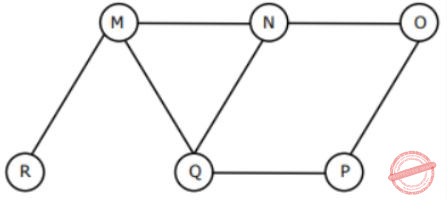A MNOPQR B NQMPOR C QMNPRO D QMNPOR
Data-Structures       Graphs
Question 19 Explanation: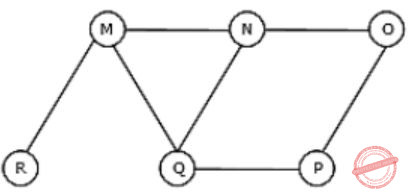Option C: QMNPRO
→ Queue starts with Q then neighbours of Q is MNP and it is matching with the given string .
→ Now , Next node to be considered is M . Neighbours of M are N, Q and R , but N and Q are already in Queue. So, R is matching with one given string
→ Now, next node to be considered is N. Neighbours of N are M, Q and O, but M and Q are already in Queue. So, O is matching with a given string.
Hence , Option C is Correct.
Similarly, check for option (D).
 Question 20

The data blocks of a very large file in the Unix file system are allocated using

 A contiguous allocation B linked allocation C indexed allocation D an extension of indexed allocation
Operating-Systems       File-System
Question 20 Explanation:
An Unix file system can utilizes an extension of indexed allocation. Because it uses direct blocks, single indirect blocks, double indirect blocks and triple indirect blocks.
 Question 21

The minimum number of equal length subintervals needed to approximateto an accuracy of atleast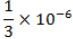using the trapezoidal rule is

 A 1000e B 1000 C 100e D 100
Engineering-Mathematics       Trapezidal Rule
Question 21 Explanation:
Note: Out of syllabus.
 Question 22

The Newton-Raphson iterationcan be used to compute the

 A square of R B reciprocal of R C square root of R D logarithm of R
Engineering-Mathematics       Newton-Raphson-Method
Question 22 Explanation:
Note: Out of syllabus.
 Question 23

Which of the following statements is true for every planar graph on n vertices?

 A The graph is connected B The graph is Eulerian C The graph has a vertex-cover of size at most 3n/4 D The graph has an independent set of size at least n/3
Engineering-Mathematics       Graph-Theory
Question 23 Explanation:
Lets do with elimination method,
(A) Consider the following disconnected graph which is planar.So false.
(B) A graph is Eulerian if all vertices have even degree but a planar graph can have vertices with odd degree.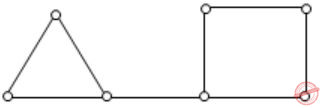So false.
(D) Consider K4 graph. It has independent set size 1 which is less than 4/3.So false.
Hence, option (C) is correct.
 Question 24

Let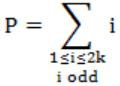and, where k is a positive integer. Then

 A P = Q - k B P = Q + k C P = Q D P = Q + 2 k
Engineering-Mathematics       Permutation-and-Combination
Question 24 Explanation:P = 1+3+5+7+...+(2k-1)
= (2-1)+(4-1)+(6-1)+(8-1)+...+(2k-1)
= (2+4+6+8+...+2k)+(-1+-1+-1+k times)
= Q-(1+1+...+k times)
= Q-k
 Question 25

A point on a curve is said to be an extremum if it is a local minimum or a local maximum. The number of distinct extrema for the curve 3x4 - 16x3 + 24x2 + 37 is

 A 0 B 1 C 2 D 3
Engineering-Mathematics       Calculus
Question 25 Explanation:
f(x) = 3x4 - 16x3 + 24x2 + 37
f’(x) = 12x3 + 48x2 + 48x = 0
12x(x2 - 4x + 4) = 0
x=0; (x-2)2 = 0
x=2
f’’(x) = 36x2 - 96x + 48
f ”(0) = 48
f ”(2) = 36(4) - 96(2) + 48
= 144 - 192 + 48
= 0
At x=2, we can’t apply the second derivative test.
f’(1) = 12; f’(3) = 36, on either side of 2 there is no sign change then this is neither minimum or maximum.
Finally, we have only one Extremum i.e., x=0.
 Question 26

If P, Q, R are Boolean variables, then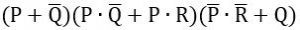Simplifies to

 ABC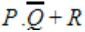D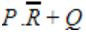Digital-Logic-Design       Boolean-Algebra
Question 26 Explanation:Question 27

Aishwarya studies either computer science or mathematics everyday. If she studies computer science on a day, then the probability that she studies mathematics the next day is 0.6. If she studies mathematics on a day, then the probability that she studies computer science the next day is 0.4. Given that Aishwarya studies computer science on Monday, what is the probability that she studies computer science on Wednesday?

 A 0.24 B 0.36 C 0.4 D 0.6
Engineering-Mathematics       Probability
Question 27 Explanation:
→ Aishwarya studied CS on Monday. Then we have two possibilities to she study computer science on wednesday.
(i) She study Mathematics on Tuesday and computer science on wednesday.
⇒ 0.6×0.4
⇒ 0.24
(ii) She study computer science on Tuesday and computer science on wednesday.
⇒ 0.4×0.4
⇒ 0.16
→ The probability that she study computer science on wednesday is
0.24+0.16 = 0.40
 Question 28

How many of the following matrices have an eigenvalue 1?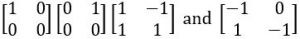A one B two C three D four
Engineering-Mathematics       Linear-Algebra
Question 28 Explanation: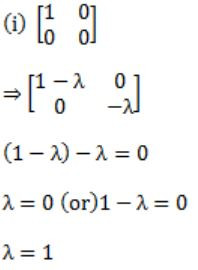Answer: We have only one matrix with eigen value 1.
 Question 29

Let X be a random variable following normal distribution with mean +1 and variance 4. Let Y be another normal variable with mean -1 and variance unknown. If P(X ≤ -1) = P(Y ≥ 2), the standard deviation of Y is

 A 3 B 2 C √2 D 1
Engineering-Mathematics       Probability
Question 29 Explanation:
P(X ≤ -1) = P(Y ≥ 2)
We can compare their values using standard normal distributions.The above equation satisfies when σy will be equal to 3.
 Question 30

Let fsa and pda be two predicates such that fsa(x) means x is a finite state automaton, and pda(y) means that y is a pushdown automaton. Let equivalent be another predicate such that equivalent (a, b) means a and b are equivalent. Which of the following first order logic statements represents the following:

Each finite state automaton has an equivalent pushdown automaton

 A (∀x fsa(x)) ⇒ (∃y pda(y) ∧ equivalent(x,y)) B ∼∀y(∃x fsa(x) ⇒ pda(y) ∧ equivalent(x,y)) C ∀x ∃y(fsa(x) ∧ pda(y) ∧ equivalent(x,y)) D ∀x ∃y(fsa(y)∧ pda(x) ∧ equivalent(x,y))
Engineering-Mathematics       Propositional-Logic
Question 30 Explanation:
Go through the options.
Option A:
If everything is a FSA. Then there exists an equivalent PDA for everything.
Option B:
Not for the case Y, if there exists a FSA then it can have equivalent PDA.
Option C:
Everything is a PDA and consists equivalent PDA.
Option D:
Everything is a PDA and has exist an equivalent FSA. In option A we are getting the equivalent of a and b.
So answer is option A.
 Question 31

P and Q are two propositions. Which of the following logical expressions are equivalent?

I. P∨∼Q
II. ∼(∼P∧Q)
III. (P∧Q)∨(P∧∼Q)∨(∼P∧∼Q)
IV. (P∧Q)∨(P∧∼Q)∨(∼P∧Q)
 A Only I and II B Only I, II and III C Only I, II and IV D All of I, II, III and IV
Engineering-Mathematics       Propositional-Logic
Question 31 Explanation:
I. P∨∼Q (✔️)
II. ∼(∼P∧Q)⇒(P∨∼Q)≡I (✔️)
III. (P×Q)∨(P×∼Q)∨(∼P×∼Q)
P∧(Q∨∼Q)∨(∼P∧∼Q)
P∨(∼P×∼Q)
(P∨∼P)×(P∨∼Q)
(P∨∼Q)≡I=II (✔️)
IV. (P×Q)∨(P∧∼Q)∨(∼P×Q)
P×(Q∨∼Q)∨(∼P∧Q)
P∨(∼P×Q)
(P∨∼P)×(P∨Q)
(P∨Q)≠I (❌)
So I≡II≡III (✔️)
 Question 32

For a magnetic disk with concentric circular tracks, the seek latency is not linearly proportional to the seek distance due to

 A non-uniform distribution of requests B arm starting and stopping inertia C higher capacity of tracks on the periphery of the platter D use of unfair arm scheduling policies
Computer-Organization       Secondary-Storage
Question 32 Explanation:
Whenever the head moves from one track to another track its speed changes, this is the case of inertia .
Because the definition of inertia is a property of matter by which it continues in its existing state of rest or uniform motion in a straight line, unless that state is changed by an external force.
 Question 33

Which of the following is/are true of the auto-increment addressing mode?

I. It is useful in creating self-relocating code
II. If it is included in an Instruction Set Architecture, then an additional ALU is required for effective address calculation
III. The amount of increment depends on the size of the data item accessed
 A I only B II only C III Only D II and III only
Computer-Organization       Addressing-Modes
Question 33 Explanation:
I. Self relocating code always takes some address in memory. So auto-increment mode is not used for self relocating code. Hence this statement is wrong.
II. An additional ALU is not necessary for auto-increment. So this statement is wrong.
III. In auto-increment addressing mode the address where next data block to be stored is generated automatically depending upon the size of single data item required to store. This is based on pointer arithmetic. So this statement is true.
Hence option C is the answer.
 Question 34

Which of the following must be true for the RFE (Return From Exception) instruction on a general purpose processor?

I. It must be a trap instruction
II. It must be a privileged instruction
III. An exception cannot be allowed to occur during execution of an RFE instruction
 A I only B II only C I and II only D I, II and III only
Computer-Organization       Machine-Instructions
Question 34 Explanation:
RFE is a privileged instruction that is performed explicitly by the Operating System to switch from kernel mode to user mode at the end of handling an exception. Hence it has to be a trap. We know when a trap/interrupt is in execution, till its completion all other trap/interrupts will not be allowed to execute. So option D is correct answer.
 Question 35

For inclusion to hold between two cache levels L1 and L2 in a multi-level cache hierarchy, which of the following are necessary?

I. L1 must be a write-through cache
II. L2 must be a write-through cache
III. The associativity of L2 must be greater than that of L1
IV. The L2 cache must be at least as large as the L1 cache
 A IV only B I and IV only C I, III and IV only D I, II, III and IV
Computer-Organization       Cache
Question 35 Explanation:
Inclusion property: in this the presence of an address in a given level of the memory system guarantees that the address is present in all lower levels of the memory system.
1st is not always correct as data need not to be exactly same at the same point of time and so write back policy can be used in this instead of write through policy.
2nd is not needed when talking only about L1 and L2, as whether L2 is write through will have impact on any memory higher than L2, not on L1.
For 3rd, associativity can be equal also, so it need not be true.
For 4th statement, L2 cache has to be as large as L1 cache. In most cases L2 cache will be generally larger than L1 cache, but we will never have an L2 cache smaller than L1 cache. So only 4th statement is necessarily true. Hence option A is the answer.
 Question 36

Which of the following are NOT true in a pipelined processor?

I. Bypassing can handle all RAW hazards
II. Register renaming can eliminate all register carried WAR hazards
III. Control hazard penalties can be eliminated by dynamic branch prediction
 A I and II only B I and III only C II and III only D I, II and III
Computer-Organization       Pipelining
Question 36 Explanation:
I. False. Bypassing can't handle all RAW hazard.
II. True. Register renaming can eliminate all WAR Hazard as well as WAW hazard.
III. If this statement would have said that
"Control hazard penalties can be completely eliminated by dynamic branch prediction", then it is false. But it is only given that "Control hazard penalties can be eliminated by dynamic branch prediction". So, it is true.
Hence, none of the given Option is Correct.
 Question 37

The use of multiple register windows with overlap causes a reduction in the number of memory accesses for

I. Function locals and parameters
II. Register saves and restores
III. Instruction fetches
 A I only B II only C III only D I, II and III
Computer-Organization       Run-Time-Environments
Question 37 Explanation:
I is true because when we make a function call there are some input registers and some output registers. If function F() is calling function G(), we can make the caller function F()'s output registers the same as the called procedure G()'s input registers this is done using overlapping register windows.This will reduce the memory accesses so that F()'s output need not be put into memory for G() to access again from memory.
II is false as register saves and restores would still be required for each and every variable.
III is also false as instruction fetch is not affected by memory access using multiple register windows.
 Question 38

In an instruction execution pipeline, the earliest that the data TLB (Translation Lookaside Buffer) can be accessed is

 A Before effective address calculation has started B During effective address calculation C After effective address calculation has completed D After data cache lookup has completed
Computer-Organization       Virtual Memory
Question 38 Explanation:
TLB is a mini page table and it contains the frequently accessed page table entries. Because logical address is effective address, only after we calculate what is the effective address we can access the TLB. Hence option C is the correct answer.
 Question 39

Consider the following functions:

f (n) = 2n
g(n) = n!
h (n) = nlogn

Which of the following statements about the asymptotic behaviour of f(n), g(n), and h(n) is true?

 A f(n) = O(g(n)); g(n) = O(h(n)) B f(n) = Ω(g(n)); g(n) = O(h(n)) C g(n) = O(f(n)); h(n) = O(f(n)) D h(n) = O(f(n)); g(n) = Ω(f(n))
Algorithms        Time-Complexity
Question 39 Explanation:
When we want to find asymptotically bigger/smaller functions, we have to follow basically 2 steps:
1. Check whether the function if polynomial time or exponential time.
2. Group them into polynomial functions and exponential function. The exponential functions are always bigger than polynomial functions.
As per the above 3 functions, the g(n) is greater than others. f(n) greater than h(n). So, the order of growth h(n) < f(n) < g(n).
Note: For computing, take always big number.
 Question 40

The minimum number of comparisons required to determine if an integer appears more than n/2 times in a sorted array of n integers is

 A θ(n) B θ(logn) C θ(log*n) D θ(1)
Algorithms        Time-Complexity
Question 40 Explanation:
The Best way to find out whether an integer appears more than n/2 times in a sorted array(Ascending Order) of n integers, would be binary search approach.
1. The First occurrence of an element can be found out in O(log(n)) time using divide and conquer technique, lets say it is i.
2. The Last occurrence of an element can be found out in O(log(n)) time using divide and conquer technique,lets say it is j.
3. Now number of occurrence of that element(count) is (j-i+1).
Overall time complexity = log n +log n +1 = O(logn)
Program:
/* If x is present in arr[low...high] then returns the index of first occurrence of x, otherwise returns -1 */
int binarySearch(int arr[], int low, int high, int x){
if (high >= low) {
int mid = (low + high)/2; /*low + (high - low)/2;*/
/* Check if arr[mid] is the first occurrence of x.
arr[mid] is first occurrence if x is one of the following is true:
(i) mid == 0 and arr[mid] == x
(ii) arr[mid-1] < x and arr[mid] == x */
if ( (mid == 0 || x > arr[mid-1]) && (arr[mid] == x) )
return mid;
else if (x > arr[mid]
) return_binarySearch(arr, (mid + 1), high, x);
else
return_binarySearch(arr, low, (mid - 1), x);
}
return -1;
}
Note: The code finds out the first occurrence of the element in the array and checks if the element at (n/2+1)th position is same(as the array is sorted). Takes O(logn) time.
 Question 41

A B-tree of order 4 is built from scratch by 10 successive insertions. What is the maximum number of node splitting operations that may take place?

 A 3 B 4 C 5 D 6
Database-Management-System       B-Trees
Question 41 Explanation:
Let's take 10 successive key values,
{1,2,3,...,10} which can cause maximum possi ble splits.
1, 2, 3 →4 →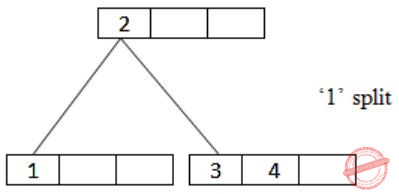5 →6 →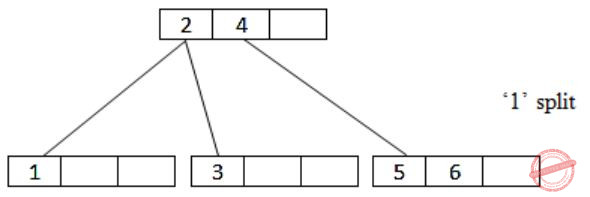7 →8 →9 →10 →Question 42

G is a graph on n vertices and 2n–2 edges. The edges of G can be partitioned into two edge-disjoint spanning trees. Which of the following is NOT true for G?

 A For every subset of k vertices, the induced subgraph has at most 2k–2 edges B The minimum cut in G has at least two edges C There are two edge-disjoint paths between every pair to vertices D There are two vertex-disjoint paths between every pair of vertices
Data-Structures       Graphs
Question 42 Explanation:
→ In Spanning tree n nodes require n-1 edges. The above question they mentioned 2 disjoint spanning trees. So, it requires n-1 + n-1 = 2n-2 edges. Except option D everything is correct.
> Option A: True: Subgraph with k vertices here is no chance to get more than 2k−2 edges. Subgraph with n−k vertices, definitely less than 2n−2k edges.
-> Option B: True: Take any subgraph SG with k vertices. The remaining subgraph will have n−k vertices. Between these two subgraphs there should be at least 2 edges because we are taken 2 spanning trees in SG.
-> Option C: True: A spanning tree covers all the vertices. So, 2 edge-disjoint spanning trees in G means, between every pair of vertices in G we have two edge-disjoint paths (length of paths may vary).
 Question 43

Consider the Quicksort algorithm. Suppose there is a procedure for finding a pivot element which splits the list into two sub-lists each of which contains at least one-fifth of the elements. Let T(n) be the number of comparisons required to sort n elements. Then

 A T(n) ≤ 2T(n/5) + n B T(n) ≤ T(n/5) + T(4n/5) + n C T(n) ≤ 2T(4n/5) + n D T(n) ≤ 2T(n/2) + n
Algorithms       Sorting
Question 43 Explanation:
Consider the case where one subset of set of n elements contains n/5 elements and another subset of set contains 4n/5 elements.
So, T(n/5) comparisons are needed for the first subset and T(4n/5) comparisons needed for second subset.
Now, suppose that one subset contains more than n/5 elements then another subset will contain less than 4n/5 elements. Due to which time complexity will be less than
T(n/5) + T(4n/5) + n
Because recursion tree will be more balanced.
 Question 44

The subset-sum problem is defined as follows: Given a set S of n positive integers and a positive integer W, determine whether there is a subset of S Whose elements sum to

An algorithm Q solves this problem in O(nW) time. Which of the following statements is false?

 A Q solves the subset-sum problem in polynomial time when the input is encoded in unary B Q solves the subset-sum problem in polynomial time when the input is encoded in binary C The subset sum problem belongs to the class NP D The subset sum problem is NP-hard
Algorithms       P-NP
Question 44 Explanation:
Note: Out of syllabus.
 Question 45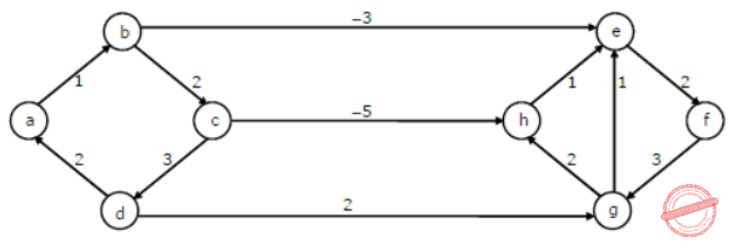Dijkstra’s single source shortest path algorithm when run from vertex a in the above graph, computes the correct shortest path distance to

 A only vertex a B only vertices a, e, f, g, h C only vertices a, b, c, d D all the vertices
Algorithms       Shortest-Path
Question 45 Explanation:
The single source shortest path algorithm(Dijkstra’s) is not work correctly for negative edge weights and negative weight cycle. But as per the above graph it works correct. It gives from shortest path between ‘a’ vertex to all other vertex. The Dijkstra’s algorithm will follow greedy strategy.
A - B ⇒ 1
A - C ⇒ 1 + 2 = 3
A - E ⇒ 1 - 3 = -2
A - F ⇒ 1 -3 + 2 = 0
A - G ⇒ 1 - 3 + 2 + 3 = 3
A - C ⇒ 1 + 2 = 3
A - H ⇒ 1 + 2 - 5 = -2
 Question 46

You are given the postorder traversal, P, of a binary search tree on the n elements 1, 2, ..., n. You have to determine the unique binary search tree that has P as its postorder traversal. What is the time complexity of the most efficient algorithm for doing this?

 A θ(log n) B θ(n) C θ(nlog n) D None of the above, as the tree cannot be uniquely determined
Data-Structures       Binary-Search-Tree
Question 46 Explanation:
Last element in post order is the root of tree- find this element in inorder-log n time. Now as in quick sort consider this as pivot and split the post order array into 2. All elements smaller than pivot goes to left and all elements larger than pivot goes to right and suppose we have k elements smaller than pivot, these elements will be same in both in-order as well as post order. Now, we already got the root, now left child is the left split and right child is the right split.
T(n) = T(k) + T(n-k-1) + logn
In worst case T(n) = O(nlogn), when k=0
But searching for an element in the in-order traversal of given BST can be done in O(1) because we have n elements from 1...n so there is no need to search an element- if last element in post order is say 5 we take it as root and since 4 elements are split the post order array into two (first 4 elements), (6th element onward) and solve recursively. Time complexity for this would be
T(n) = T(k) + T(n-k-1)+O(1)
Which gives T(n) = O(n)
since we know that all elements must be traversed at least once T(n) = θ(n)
 Question 47

We have a binary heap on n elements and wish to insert n more elements (not necessarily one after another) into this heap. The total time required for this is

 A θ(log n) B θ(n) C θ(nlog n) D θ(n2)
Data-Structures       Heap-Tree
Question 47 Explanation:
Inserting an element into binary(either max or min) heap takes O(logn) for all cases, but in question they clearly mentioned that n elements and inserting one by one n elements, so it takes 2n time. So, O(n).
Note: We can also insert all the elements once, there will be no difference on time complexity.
 Question 48

Which of the following statements is false?

 A Every NFA can be converted to an equivalent DFA B Every non-deterministic Turing machine can be converted to an equivalent deterministic Turing machine C Every regular language is also a context-free language D Every subset of a recursively enumerable set is recursive
Theory-of-Computation       Recursive-Enumerable-Languages
Question 48 Explanation:
Every NFA can be converted into DFA (as there exist a standard procedure to convert NFA into DFA). Also, every non-deterministic Turing machine can be converted to an equivalent deterministic Turing machine. Every regular language is also a CFL , since if a language can be recognized by Finite automata, then it must also be recognize by PDA (as PDA is more powerful than FA). But every subset of recursively enumerable need not be recursive.
 Question 49

Given below are two finite state automata (→ indicates the start state and F indicates a final state)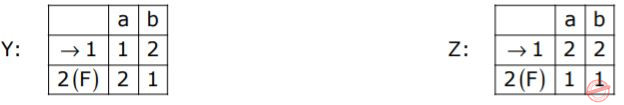Which of the following represents the product automaton Z×Y?

 A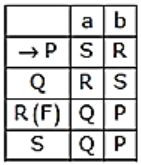B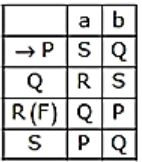CDTheory-of-Computation       Finite-Automata
Question 49 Explanation:
The product automata will have states {11, 12, 21, 22} and “11” is inItial state and “22” is final state. By comparison we can easily infer that state {11, 12, 21, 22} is renamed as {P, Q, S, R}, where P is initial state (state “11”) and R is final state (state “22”).
Lets rename table Z (for sake of clarity)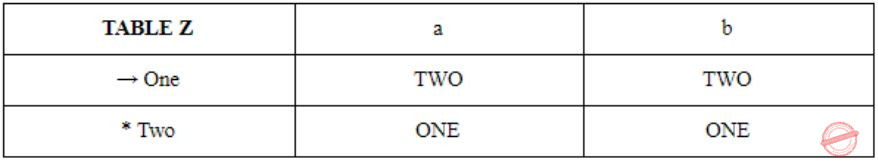And Table Y (same as given in question)The product automata will have states { One1, One2, Two1, Two2} Where One1 is P , Two2 is R and One2 is Q and Two1 is S.
The transition table for Z × Y is given below:NOTE: LAST TWO ROWS DOESN’T MATCH WITH OPTION A. BUT IF THE ASSUMPTION IN QUESTION SUCH AS STATE “11” IS P AND STATE “22” IS R, HOLDS, THEN THE ONLY OPTION MATCHES WITH PRODUCT AUTOMATA IS OPTION A, AS (FIRST ROW) , P (ON “a”) -> S AND P (ON “b”) -> R, IS THE ONLY OPTION MATCHING WITH OPTION A.
 Question 50

Which of the following statements are true?

I. Every left-recursive grammar can be converted to a right-recursive grammar and vice-versa
II. All \epsilon productions can be removed from any context-free grammar by suitable transformations
III. The language generated by a context-free grammar all of whose productions are of the form X → w or X → wY (where, w is a string of terminals and Y is a non-terminal), is always regular
IV. The derivation trees of strings generated by a context-free grammar in Chomsky Normal Form are always binary trees
 A I, II, III and IV B II, III and IV only C I, III and IV only D I, II and IV only
Theory-of-Computation       Contest-Free-Grammar
Question 50 Explanation:
Every left recursive grammar can be converted to a right-recursive grammar and vice-versa, the conversion of a left recursive grammar into right recursive is also known as eliminating left recursion from the grammar.
Statement III is also true, as this is the standard definition of regular grammar (TYPE 3 grammar).
Statement IV is also true, as in CNF the only productions allowed are of type:
A-> BC
A-> a // where “a” is any terminal and B, C are any variables.
When we draw the parse tree for the grammar in CNF, it will always have at most two childs in every step, so it always results binary tree.
But statement II is false, as if the language contains empty string then we cannot remove every epsilon production from the CFG, since at least one production (mainly S → ϵ) must be there in order to derive empty string in language.
 Question 51

Match the following:A E - P, F - R, G - Q, H - S B E - R, F - P, G - S, H - Q C E - R, F - P, G - Q, H - S D E - P, F - R, G - S, H - Q
Theory-of-Computation       Match-the-Following
Question 51 Explanation:
The grammar in S {X →bXb | cXc | ϵ} derives all even length Palindromes, So H matches with S.
F matches with P, Number of formal parameters in the declaration…. matches with {L = { an bm c n dm | m,n >=1}
Since, an bm corresponds to formal parameter (if n=2 and m=1, and “a” is int type and “b”is float type, then it means (int,int,float)) and cn dm corresponds to actual parameter used in function.
Similarly other two can also be argued by their reasons, but with F matches with P and H matches with S implies that option C is the only correct option.
 Question 52

Match the following NFAs with the regular expressions they correspond to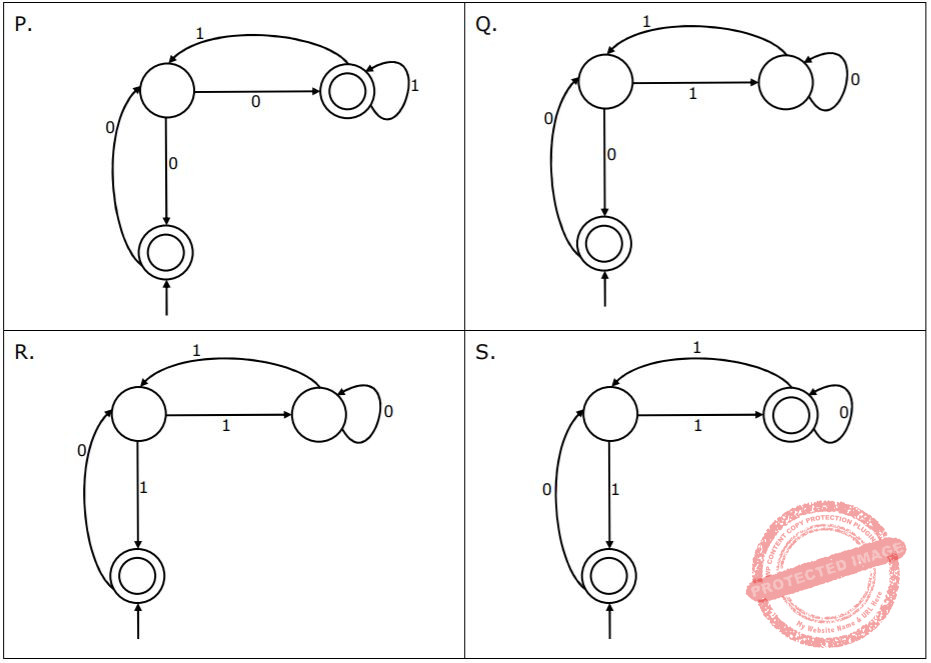1. ϵ + 0(01*1 + 00) * 01*
2. ϵ + 0(10 *1 + 00) * 0
3. ϵ + 0(10 *1 + 10) *1
4. ϵ + 0(10 *1 + 10) *10 *
 A P-2, Q-1, R-3, S-4 B P-1, Q-3, R-2, S-4 C P-1, Q-2, R-3, S-4 D P-3, Q-2, R-1, S-4
Theory-of-Computation       Finite-Automata
Question 52 Explanation:
The NFA represented by P, accepts string “00” and then at final state (other than initial state) we have self loop of “1” , so we conclude that it must accept the string of the form of -> ϵ + 0 X* 01*, where X is regular expression (01*1 + 00) {resolving the loop at middle state}. It matches with statement 1.
Similarly, The NFA represented by Q, has the form of -> ϵ + 0X*0, where X is regular expression (10*1 + 00) {resolving the loop at middle state}. It matches with statement 2.
The NFA represented by R, has the form of -> ϵ + 0X*1, where X is regular expression (10*1 + 01) {resolving the loop at middle state}. It matches with statement 3.
The NFA represented by S, accepts string “01” and then at final state (other than initial state) we have self loop of “0”, so we conclude that it must accept the string of the form of -> ϵ + 0X* 10*, where X is regular expression (10*1 + 10) {resolving the loop at middle state}. It matches with statement 4.
 Question 53

Which of the following are regular sets?

I. {anb2m | n≥0, m≥0}
II. {anbm | n=2m}
III. {anbm | n≠2m}
IV. {xcy | x,y∈{a,b}*}
 A I and IV only B I and III only C I only D IV only
Theory-of-Computation       Regular Languages
Question 53 Explanation:
Statement I represents a regular language whose regular expression is a* (bb)*. Also it doesn’t require any comparison between “a” and “b” , so it can be recognized by DFA and hence regular.
Statement II and III represent CFL, as it requires comparison between number of a’s and b’s.
Statement IV is also regular, and its regular expression is (a+b)* c (a+b)*.
 Question 54

Which of the following are true?

I. A programming language which does not permit global variables of any kind and has no nesting of procedures/functions, but permits recursion can be implemented with static storage allocation
II. Multi-level access link (or display) arrangement is needed to arrange activation records only if the programming language being implemented has nesting of procedures/functions
III. Recursion in programming languages cannot be implemented with dynamic storage allocation
IV. Nesting procedures/functions and recursion require a dynamic heap allocation scheme and cannot be implemented with a stack-based allocation scheme for activation records
V.Programming languages which permit a function to return a function as its result cannot be implemented with a stack-based storage allocation scheme for activation records
 A II and V only B I, III and IV only C I, II and V only D II, III and V only
Compiler-Design       Run-Time-Environments
Question 54 Explanation:
II. Multilevel access link (or display) arrangement is needed to arrange Activation Records only if the programming language being implemented has nesting of procedures and functions.
V. PL’s which permits a function to return a function as its result cannot be implemented with a stack-based storage allocation scheme for activation records.
II & V are True.
 Question 55

An LALR(1) parser for a grammar G can have shift-reduce (S-R) conflicts if and only if

 A the SLR(1) parser for G has S-R conflicts B the LR(1) parser for G has S-R conflicts C the LR(0) parser for G has S-R conflicts D the LALR(1) parser for G has reduce-reduce conflicts
Compiler-Design       Parsres
Question 55 Explanation:
LALR(1) parser is obtained by minimizing the LR(1) parser and hence they both uses LR(1) items. Now consider if LR(1) parser has SR conflict, for ex:
Consider a state in LR(1) parser:
S-> x.yA, a
A-> x. , y
This has both shift and reduce conflict on symbol “y”.
Since LR(1) already has SR conflict , so resulting LALR(1) (after merging) will also have SR conflict.
Now if LR(1) doesn’t have SR conflict then it is guaranteed that the LALR(1) will never have SR conflict. The reason behind this is, as we merge those state only which has same set of canonical items except look ahead and the LR(1) canonical items has DFA (means from one state to other state the transition is from unique symbol) , so after merging also we will never see any shift conflict, only reduce-reduce may occur.
Hence An LALR(1) parser for a grammar G can have shift-reduce (S-R) conflicts if and only if the LR(1) parser for G has S-R conflicts.
 Question 56

In the slow start phase of the TCP congestion control algorithm, the size of the congestion window

 A does not increase B increases linearly C increases quadratically D increases exponentially
Computer-Networks
Question 56 Explanation:
In slow start phase, window size will grow exponentially, when the threshold is reached and congestion avoidance phase begins. In congestion avoidance phase, the window is increased linearly.
 Question 57

If a class B network on the Internet has a subnet mask of 255.255.248.0, what is the maximum number of hosts per subnet?

 A 1022 B 1023 C 2046 D 2047
Computer-Networks       IP-Address
Question 57 Explanation:
255.255.248.0 can be written as 11111111.11111111.11111000.00000000
Number of bits assigned for host id is the number of zeros in subnet mask. Here 11 bits are used.
for host id so maximum possible hosts are= 211 - 2 = 2046
 Question 58

A computer on a 10Mbps network is regulated by a token bucket. The token bucket is filled at a rate of 2Mbps. It is initially filled to capacity with 16Megabits. What is the maximum duration for which the computer can transmit at the full 10Mbps?

 A 1.6 seconds B 2 seconds C 5 seconds D 8 seconds
Computer-Networks       Token-Bucket
Question 58 Explanation:
Duration = C/x-y, where C is the initial capacity, x is outgoing rate and y is incoming rate.
 Question 59

A client process P needs to make a TCP connection to a server process S. Consider the following situation: the server process S executes a socket (), a bind () and a listen () system call in that order, following which it is preempted. Subsequently, the client process P executes a socket () system call followed by connect () system call to connect to the server process S. The server process has not executed any accept() system call. Which one of the following events could take place?

 A connect ( ) system call returns successfully B connect ( ) system call blocks C connect ( ) system call returns an error D connect ( ) system call results in a core dump
Computer-Networks       Sockets
Question 59 Explanation:
Connect() System call is not blocking system call but it blocks until connection is established or rejected. If accept() is not executed at server then connection will be rejected and an error statement is returned.
 Question 60

What is printed by the following C program?

int f(int x, int *py, int **ppz)           void main()
{                                               {
int y, z;                                      int c, *b, **a;
**ppz += 1; z = **ppz;                         c = 4; b = &c; a = &b;
*py += 2; y = *py;                             printf("%d",f(c,b,a));
x += 3;                                       }
return x + y + z;
}  
 A 18 B 19 C 21 D 22
Programming       C-Programming
Question 60 Explanation:
f(c,b,a)
f(c,&c,&(&c)) = f(4,4,4)
c is 4, b is a pointer pointing address of a, a is a pointer to pointer of c. Hence both b and c are pointing to same memory address i.e., a.
Hence whatever increment operation happens in f, it happens/ reflects on same value i.e., a.
**ppz+=1;
z=**ppz; //z=5
These steps update it to 5 and stored in z.
*py+=2; //changes c to 7, x is unchanged.
y=*py; //y=7
It updates to 7 and stored in y.
x+=3 //x is incremented by 3.
returns x+y+z = 7+7+5 = 19
 Question 61

Choose the correct option to fill ?1 and ?2 so that the program below prints an input string in reverse order. Assume that the input string is terminated by a newline character.

void reverse(void)
{
int c;
if(?1)reverse();
?2
}
int main(){
printf("Enter Text"); printf("n");
reverse();printf("n") ;
}
 A ?1 is (getchar( ) != ’\n’) ?2 is getchar(c); B ?1 is (c = getchar( ) ) != ’\n’) ?2 is getchar(c); C ?1 is (c != ’\n’) ?2 is putchar(c); D ?1 is ((c = getchar()) != ’\n’) ?2 is putchar(c);
Programming       C-Programming
Question 61 Explanation:
void reverse(void)
{
int c;
if(?1) reverse( );
?2
}
main( )
{
printf(“Enter Text”);
printf(“\n”);
reverse( );
printf(“\n”);
}
We can simply eliminate A & B for ?2.
& Hence
?1 is ((c=getchar( )) != ‘\n’)
?2 is putchar(c);
 Question 62

The following C function takes a single-linked list of integers as a parameter and rearranges the elements of the list. The function is called with the list containing the integers 1, 2, 3, 4, 5, 6, 7 in the given order. What will be the contents of the list after the function completes execution?

struct node {
int value;
struct node *next;
};
void rearrange(struct node *list) {
struct node *p, * q;
int temp;
if ((!list) || !list->next)return;
p = list; q = list->next;
while(q) {
temp = p->value;p->value = q->value;
q->value = temp;p = q->next;
q = p?p->next:0;
}
}
 A 1,2,3,4,5,6,7 B 2,1,4,3,6,5,7 C 1,3,2,5,4,7,6 D 2,3,4,5,6,7,1
Data-Structures       Linked-List
Question 62 Explanation:
The given list is 1,2,3,4,5,6,7.
After 1st Iteration: 2,1,3,4,5,6,7
2nd Iteration: 2,1,4,3,5,6,7
3rd Iteration: 2,1,4,3,6,5,7
After each exchange is done, it starts from unchanged elements due to p=q⟶next;
‘p’ pointing to Null & q pointing to 7.
Hence 2,1,4,3,6,5,7.
 Question 63

The P and V operations on counting semaphores, where s is a counting semaphore, are defined as follows:

P(s): s =  s - 1;
if (s < 0) then wait;
V(s): s = s + 1;
if (s <= 0) then wakeup a process waiting on s;

Assume that Pb and Vb the wait and signal operations on binary semaphores are provided. Two binary semaphores Xb and Yb are used to implement the semaphore operations P(s) and V(s) as follows:

P(s): Pb(Xb);
s = s - 1;
if (s < 0) {
Vb(Xb) ;
Pb(Yb) ;
}
else Vb(Xb);

V(s): Pb(Xb) ;
s = s + 1;
if (s <= 0) Vb(Yb) ;
Vb(Xb) ;

The initial values of Xb and Yb are respectively

 A 0 and 0 B 0 and 1 C 1 and 0 D 1 and 1
Operating-Systems       Process-Synchronization
Question 63 Explanation:
xb must be 1 because both P(s) operation and V(s) operation perform Pb(xb) first. So if xb=0, then all the process performing these operations will be blocked.
Yb must be '0' initially, because if Yb is '1' initially then two process can be in critical section at the same time.
So answer is Option (C).
 Question 64

Which of the following statements about synchronous and asynchronous I/O is NOT true?

 A An ISR is invoked on completion of I/O in synchronous I/O but not in asynchronous I/O B In both synchronous and asynchronous I/O, an ISR (Interrupt Service Routine) is invoked after completion of the I/O C A process making a synchronous I/O call waits until I/O is complete, but a process making an asynchronous I/O call does not wait for completion of the I/O D In the case of synchronous I/O, the process waiting for the completion of I/O is woken up by the ISR that is invoked after the completion of I/O
Operating-Systems       I/O-Handling
Question 64 Explanation:
Synchronous I/O mean that some flow of execution (such as a process or thread) is waiting for the operation to complete. Asynchronous I/O means that nothing is waiting for the operation to complete and the completion of the operation itself causes something to happen.
Synchronous I/O -- some execution vehicle (like a process or thread) that initiates the I/O also waits for the I/O to complete (and perhaps completes it). When the I/O completes, that same execution vehicle goes on to do something else, perhaps using the results of the I/O.
Asynchronous I/O -- no execution vehicle waits for the I/O to complete. When the I/O completes, whatever execution vehicle happens to complete the I/O may arrange for later things to happen.
Option B is not true, because both synchronous and asynchronous I/O, an ISR (Interrupt Service Routine) is not invoked after completion of the I/O.
 Question 65

Which of the following is NOT true of deadlock prevention and deadlock avoidance schemes?

 A In deadlock prevention, the request for resources is always granted if the resulting state is safe B In deadlock avoidance, the request for resources is always granted if the result state is safe C Deadlock avoidance is less restrictive than deadlock prevention D Deadlock avoidance requires knowledge of resource requirements a priori
Operating-Systems       Deadlock
Question 65 Explanation:
Deadlock prevention scheme handles deadlock by making sure that one of the four necessary conditions don't occur. So, it may be the case that a resource request might be rejected even if the resulting state is safe. Hence, option (A) is false.
 Question 66

A process executes the following code

for(i=0; i<n; i++) for();

The total number of child processes created is

 A n B (2n) - 1 C 2n D (2n+1) - 1
Operating-Systems       System-Calls
Question 66 Explanation:
Fork is a system call, implements in kernel.
It is a operation where process creates a copy of itself.1,3,7,15,31,... ⇒ 2n-1
 Question 67

A processor uses 36 bit physical addresses and 32 bit virtual addresses, with a page frame size of 4 Kbytes. Each page table entry is of size 4 bytes. A three level page table is used for virtual to physical address translation, where the virtual address is used as follows

• Bits 30-31 are used to index into the first level page table
• Bits 21-29 are used to index into the second level page table
• Bits 12-20 are used to index into the third level page table, and
• Bits 0-11 are used as offset within the page

The number of bits required for addressing the next level page table (or page frame) in the page table entry of the first, second and third level page tables are respectively.

 A 20, 20 and 20 B 24, 24 and 24 C 24, 24 and 20 D 25, 25 and 24
Operating-Systems       Virtual Memory
Question 67 Explanation:
Virtual address size = 32 bits
From the question we can see the below info:
Physical address size = 36 bits
Physical memory size = 236 bytes
Page frame size = 4K bytes = 212 bytes
No. of bits for offset = 12
No. of bits required to access physical memory frame = 36 – 12 = 24
So in third level of page table, 24 bits are required to access an entry.
In second level page table entry -- 9 bits of virtual address are used to access second level page table entry and size of pages in second level is 4 bytes.
So size of second level page table is (29)*4 = 211 bytes. It means there are (236)/(211) possible locations to store this page table. Therefore the second page table requires 25 bits to address it. the first page table needs 25 bits.
Answer - D
First level
 Question 68

Let R and S be two relations with the following schema

R(P,Q,R1,R2,R3)
S(P,Q,S1,S2)

Where {P, Q} is the key for both schemas. Which of the following queries are equivalent?

I. ΠP(R ⨝ S)
II. ΠP(R) ⨝ ΠP(S)
III. ΠPP,Q(R) ∩ ΠP,Q(S))
IV. ΠPP,Q(R) - (ΠP,Q(R) - ΠP,Q(S)))
 A Only I and II B Only I and III C Only I, II and III D Only I, III and IV
Database-Management-System       Relational-Algebra
Question 68 Explanation:
Natural join is based on the common columns of the two tables.
We have two common columns in 'R' and 'S' which are 'P' and 'Q'.
(I) Both P and Q are used while doing the join, i.e., both P and Q are used to filter.
(II) Q is not used here for filtering. Natural join is done on all P's from R and all P's from S. So different from option (I).
(III) Through venn diagram it can be proved that A∩B = A - (A-B).
So through above formula we can say that (III) and (IV) are equivalent.
So, finally (I), (III) and (IV) are equivalent.
 Question 69

Consider the following relational schemes for a library database:

Book(Title, Author, Catalog_ no, Publisher, Year, Price)
Collection (Title, Author, Catalog_no)

with in the following functional dependencies:

I. Title Author → Catalog_no
II. Catalog_no → Title Author Publisher Year
III. Publisher Title Year → Price

Assume {Author, Title} is the key for both schemes. Which of the following statements is true?

 A Both Book and Collection are in BCNF B Both Book and Collection are in 3NF only C Book is in 2NF and Collection is in 3NF D Both Book and Collection are in 2NF only
Database-Management-System       Normalization
Question 69 Explanation:
Given that
Book(Title, Author, Catalog_no, Publisher, Year, Price)
Collection(Title, Author, Catalog_no)
I) Title Author ⟶ Catalog_no ⟶ BCNR
II) Catalog_no ⟶ Title, Author, Publisher, Year ⟶ 3NF
III) Publisher Title Year ⟶ Price ⟶ 2NF Book’s in 2NF
Collection is in 3NF.
 Question 70

Consider a file of 16384 records. Each record is 32 bytes long and its key field is of size 6 bytes. The file is ordered on a non-key field, and the file organization is unspanned. The file is stored in a file system with block size 1024 bytes, and the size of a block pointer is 10 bytes. If the secondary index is built on the key field of the file, and a multi-level index scheme is used to store the secondary index, the number of first-level and second-level blocks in the multi-level index are respectively

 A 8 and 0 B 128 and 6 C 256 and 4 D 512 and 5
Database-Management-System       Indexing
Question 70 Explanation:
Total no. of records in a file = 16384
Record size = 32 bytes
Key size = 6 bytes
Block pointer size = 10 bytes
Block size of file system = 1024 bytes
Record (or) index entry size = 10+6 = 16 bytes
In first level no. of blocks = No. of records in a file/Block size =16384 * 16/1024 = 256
In second level, it have = 256*16 entries
In second level, no. of blocks = No. of entries/Block size = 256*16/1024 = 4
 Question 71

Consider a machine with a 2-way set associative data cache of size 64Kbytes and block size 16bytes. The cache is managed using 32 bit virtual addresses and the page size is 4Kbytes. A program to be run on this machine begins as follows:

double ARR;
int i, j;
/* Initialize array ARR to 0.0 */
for(i=0; i<1024; i++)
for(j=0; j<1024; j++)
ARR[i][j] = 0.0; 

The size of double is 8Bytes. Array ARR is located in memory starting at the beginning of virtual page 0xFF000 and stored in row major order. The cache is initially empty and no pre-fetching is done. The only data memory references made by the program are those to array ARR.

The total size of the tags in the cache directory is

 A 32Kbits B 34Kbits C 64Kbits D 68Kbits
Computer-Organization       Cache
Question 71 Explanation:
It is given that cache size = 64KB
Block size = 16 Bytes = 24 Bytes, so block offset = 4 bits
Number of blocks in the cache = 64KB / 16B = 4K
It is 2-way set associative cache, so each set contains 2 blocks.
So, number of sets = 4K / 2 = 2K = 211
So, we need 11-bits for set indexing.
Since the address is 32 bits long, number of tag bits = 32−11−4 = 17
Total size of the tag directory = No. of tag bits ×Number of cache blocks = 17×4K
= 68 Kbits
 Question 72

Consider a machine with a 2-way set associative data cache of size 64Kbytes and block size 16bytes. The cache is managed using 32 bit virtual addresses and the page size is 4Kbytes. A program to be run on this machine begins as follows:

double ARR;
int i, j;
/* Initialize array ARR to 0.0 */
for(i=0; i<1024; i++)
for(j=0; j<1024; j++)
ARR[i][j] = 0.0; 

The size of double is 8Bytes. Array ARR is located in memory starting at the beginning of virtual page 0xFF000 and stored in row major order. The cache is initially empty and no pre-fetching is done. The only data memory references made by the program are those to array ARR.

Which of the following array elements has the same cache index as ARR?

 A ARR   B ARR   C ARR   D ARR  
Computer-Organization       Cache
Question 72 Explanation:
It is given that cache size = 64KB
Block size = 16 Bytes
Number of blocks in the cache = 64KB / 16B = 4K
It is 2-way set associative cache, so each set contains 2 blocks.
So, number of sets = 4K / 2 = 2K = 211
Each element size = 8B and size of each block = 16 B
No. of elements in one block = 16/8 = 2 We know no. of elements in one row of the array = 1024. So, no. of blocks for one row of the array = 1024/2 = 512
We know there are 211 sets, and each set has two blocks.
For any element to share the same cache index as ARR, it has to belong to the same set.
ARR belongs to set-0. The next element that belongs to set-0 will have block number 2048 because 2048 mod 211 = 0.
Block number 2048 will be from row number 2048/512 = 4, because each row has 512 blocks we divide the block number with 512 to get the row number.
From the given options ARR will have the same cache index as ARR.
 Question 73

Consider a machine with a 2-way set associative data cache of size 64Kbytes and block size 16bytes. The cache is managed using 32 bit virtual addresses and the page size is 4Kbytes. A program to be run on this machine begins as follows:

double ARR;
int i, j;
/* Initialize array ARR to 0.0 */
for(i=0; i<1024; i++)
for(j=0; j<1024; j++)
ARR[i][j] = 0.0; 

The size of double is 8Bytes. Array ARR is located in memory starting at the beginning of virtual page 0xFF000 and stored in row major order. The cache is initially empty and no pre-fetching is done. The only data memory references made by the program are those to array ARR.

The cache hit ratio for this initialization loop is

 A 0% B 25% C 50% D 75%
Computer-Organization       Cache
Question 73 Explanation:
Block size = 16B and it is given that double size is 8B, so one element = 8B.
So in one block 2 elements will be stored.
To store 1024×1024 elements no. of blocks needed = 1024×1024/2 = 220/2 = 219.
In each block the first element will be a miss and second element will be a hit because on every miss that entire block is brought into the cache. So, there will be 219 hits and 219 misses. Total no. of references = no. of elements in the array = 220
⇒ hit ratio = No. of hits / Total no. of references
= 219/220 = 1/2 = 0.5
= 0.5×100 = 50%
 Question 74

Consider the following C program

int f1 (int n)
{
if(n == 0||n == 1)
return n;
else
return (2*f1(n-1)+3*f1(n-2));
}

int f2 (int n)
{
int i;
int X[N], Y[N], Z[N];

X=Y=Z=0;
X=1; Y=2; Z=3;
for(i=2; i<=n; i++) {
X[i] = Y[i-1] + Z[i-2];
Y[i] = 2*X[i];
Z[i] = 3*X[i];
}
return X[n];
}

The running time of f1(n) and f2(n) are

 A θ(n) and θ(n) B θ(2n) and θ(n) C θ(n) and θ(2n) D θ(2n) and θ(2n)
Algorithms        Time-Complexity
Question 74 Explanation:
Time complexity of f1 is given by
T(n) = T(n-1) + T(n-2) (multiplication by 2 and 3 won't affect complexity as it is a constant time operation)
T(0) = T(1) = 1
The recurrence is similar to Fibonacci series. The time complexity is O(2n)
T(n) = T(n-1) + T(n-2) + c
T(0) = 1
T(n-1) ≈ T(n-2)
But in reality, T(n-1) > T(n-2)
So to find upper bound the expression will be
T(n) = 2T(n-1) + c
= 4T(n-2) + 3c
= 8T(n-3) + 7c
= 2kT(n-k) + (2k-1)c
n-k=0 ⇒ k = n
T(n) = 2nT(0) + (2n-1)c
= 2n + (2n-1)c
⇒ T(n) = O(2n)
The time required for f2 is O(n) (because it consists of only one loop).
 Question 75

Consider the following C program

int f1 (int n)
{
if(n == 0||n == 1)
return n;
else
return (2*f1(n-1)+3*f1(n-2));
}

int f2 (int n)
{
int i;
int X[N], Y[N], Z[N];

X=Y=Z=0;
X=1; Y=2; Z=3;
for(i=2; i<=n; i++) {
X[i] = Y[i-1] + Z[i-2];
Y[i] = 2*X[i];
Z[i] = 3*X[i];
}
return X[n];
}

f1(8) and f2(8) return the values

 A 1661 and 1640 B 59 and 59 C 1640 and 1640 D 1640 and 1661
Programming       C-Programming
Question 75 Explanation:
Both functions perform same operation, so output is same, means either (B) or (C) is correct.
f1(2) = 2*f1(1) + 3*f1(0) = 2
f1(3) = 2*f1(2) + 3*f1(1) = 2*2 + 3*1 = 7
f1(4) = 2*f1(3) + 3*f1(2) = 2*7 + 3*2 = 20
f1(5) = 2*f1(4) + 3*f1(3) = 2*20 + 3*7 = 40 + 21 = 61
We can skip after this as the only remaining choice is (C).
f1(6) = 2*f1(5) + 3*f1(4) = 2*61 + 3*20 = 122 + 60 = 182
f1(7) = 2*f1(6) + 3*f1(5) = 2*182 + 3*61 = 364 + 183 = 547
f1(8) = 2*f1(7) + 3*f1(6) = 2*547 + 3*182 = 1094 + 546 = 1640
 Question 76

Delayed branching can help in the handling of control hazards

For all delayed conditional branch instructions, irrespective of whether the condition evaluates to true or false

 A The instruction following the conditional branch instruction in memory is executed. B The first instruction in the fall through path is executed. C The first instruction in the taken path is executed. D The branch takes longer to execute than any other instruction.
Computer-Organization       Pipelining
Question 76 Explanation:
In order to avoid the pipeline delay due to conditional branch instruction, a suitable instruction is placed below the conditional branch instruction such that the instruction will be executed irrespective of whether branch is taken or not and won't affect the program behaviour. Hence option A is the answer.
 Question 77

Delayed branching can help in the handling of control hazards

The following code is to run on a pipelined processor with one branch delay slot:

I1: ADD R2 ← R7+R8
I2 : SUB R4 ← R5-R6
I3 : ADD R1 ← R2+R3
I4 : STORE Memory [R4] ← [R1]
BRANCH to Label if R1 == 0 

Which of the instructions I1, I2, I3 or I4 can legitimately occupy the delay slot without any other program modification?

 A I1 B I2 C I3 D I4
Computer-Organization       Pipelining
Question 77 Explanation:
It is the method to maximize the use of the pipeline by finding and executing an instruction that can be safely executed whether the branch is taken or not. So, when a branch instruction is encountered, the hardware puts the instruction following the branch into the pipe and begins executing it. Here we do not need to worry about whether the branch is taken or not, as we do not need to clear the pipe because no matter whether the branch is taken or not, we know the instruction is safe to execute.
From the given set of instructions I3 is updating R1, and the branch condition is based on the value of R1 so I3 can’t be executed in the delay slot.
Instruction I1 is updating the value of R2 and R2 is used in I3. So I1 also can’t be executed in the delay slot.
Instruction I2 is updating R4, and at the memory location represented by R4 the value of R1 is stored. So if I2 is executed in the delay slot then the memory location where R1 is to be stored as part of I4 will be in a wrong place. Hence between I2 and I4, I2 can’t be executed after I4. Hence I2 can’t be executed in the delay slot.
Instruction I4 can be executed in the delay slot as this is storing the value of R1 in a memory location and executing this in the delay slot will have no effect. Hence option D is the answer.
 Question 78

Let xn denote the number of binary strings of length n that contain no consecutive 0s.

Which of the following recurrences does xn satisfy?

 A xn = 2xn-1 B xn = x⌊n/2⌋+1 C xn = x⌊n/2⌋+n D xn = xn-1+xn-2
Algorithms        Recurrence
Question 78 Explanation:
xn = number of binary strings of length n that contains non-consecutive 0’s.
⇒ Let us consider n=1
Possible binary strings: 0, 1
So, x1 = 2
⇒ For n = 2;
Possible strings are,
010
110
111
011
101
So, x3 = 5
⇒ For n=4,
Possible strings are
0 1 1 1
1 0 1 1
1 1 0 1
1 1 1 0
0 1 1 0
0 1 0 1
1 0 1 0
1 1 1 1

So,
x4=8,
Hence, for all values of x4 above only option (D) satisfies.
 Question 79

Let xn denote the number of binary strings of length n that contain no consecutive 0s.

The value of x5 is

 A 5 B 7 C 8 D 16
Algorithms        Recurrence
Question 79 Explanation:
From above question we have found that equation,
xn = xn-1 + xn-2
satisfies.
And also we have found the value of x4 and x3 for the above equation.
So, according to the equation the value of x5 will be,
x5 = x3 + x4
= 5 + 8
= 13
Hence , none of the given option is correct.
 Question 80

The subset-sum problem is defined as follows. Given a set of n positive integers, S = {a1,a2,a3,...,an} and positive integer W, is there a subset of S whose elements sum to W? A dynamic program for solving this problem uses a 2-dimensional Boolean array X, with n rows and W+1 columns. X[i,j],1≤i≤n,0≤j≤W, is TRUE if and only if there is a subset of {a1,a2,...,ai} whose elements sum to j.

Which of the following is valid for 2≤i≤n and ai≤j≤W?

 A X[i, j] = X[i-1, j] ∨ X[i, j - ai] B X[i, j] = X[i-1, j] ∨ X[i-1, j - ai] C X[i, j] = X[i-1, j] ∧ X[i, j - ai] D X[i, j] = X[i-1, j] ∧ X[i -1, j - ai]
Algorithms        Dynamic-Programming
Question 80 Explanation:
X[i, j] (2≤i≤n and ai≤j≤W) is true if any of the following is true.
1) Sum of weights excluding ai is equal to j, i.e., if X[i-1, j] is true.
2) Sum of weights including ai is equal to j, i.e., if X[i-1, j-ai] is true so that we get (j – ai) + ai as j.
 Question 81

The subset-sum problem is defined as follows. Given a set of n positive integers, S = {a1,a2,a3,...,an} and positive integer W, is there a subset of S whose elements sum to W? A dynamic program for solving this problem uses a 2-dimensional Boolean array X, with n rows and W+1 columns. X[i,j],1≤i≤n,0≤j≤W, is TRUE if and only if there is a subset of {a1,a2,...,ai} whose elements sum to j.

Which entry of the array X, if TRUE, implies that there is a subset whose elements sum to W?

 A X[1, W] B X[n, 0] C X[n, W] D X[n-1, n]
Algorithms        Dynamic-Programming
Question 81 Explanation:
The last row and last column given the value is 1 then subset of whose elements sum to W.
Note: As per present GATE syllabus, this concept is not required.
 Question 82

Consider the following ER diagramThe minimum number of tables needed to represent M, N, P, R1, R2 is

 A 2 B 3 C 4 D 5
Database-Management-System       ER-Model
Question 82 Explanation:
➝ M, P are entities so they require individual tables.
➝ Here N is a Weak entity, but it need to modify the primary key of P such as P1
M = {M1, M2, M3, P1}
P = {P1, P2}
N = {N1, N2, P1}
➝ Relationship set has its own attribute, then no need to create a separate table.
➝ Finally we require 3 minimum tables to represent M, P, N, R1, R2.
 Question 83

Consider the following ER diagramWhich of the following is a correct attribute set for one of the tables for the correct answer to the above question?

 A {M1, M2, M3, P1} B {M1, P1, N1, N2} C {M1, P1, N1} D {M1, P1}
Database-Management-System       ER-Model
Question 83 Explanation:
Possible set of attributes is
For M = {M1, M2, M3, P1}
P = {P1, P2}
N = {N1, N2, P1}
 Question 84

Consider the following C program that attempts to locate an element x in an array Y[] using binary search. The program is erroneous.

1.   f(int Y, int x) {
2.     int i, j, k;
3.     i = 0; j = 9;
4.     do {
5.             k =  (i + j) /2;
6.             if( Y[k] < x)  i = k; else j = k;
7.         } while(Y[k] != x && i < j);
8.     if(Y[k] == x) printf ("x is in the array ") ;
9.     else printf (" x is not in the array ") ;
10. }

On which of the following contents of Y and x does the program fail?

 A Y is [1 2 3 4 5 6 7 8 9 10] and x < 10 B Y is [1 3 5 7 9 11 13 15 17 19] and x < 1 C Y is [2 2 2 2 2 2 2 2 2 2] and x > 2 D Y is [2 4 6 8 10 12 14 16 18 20] and 2 < x < 20 and x is even
Algorithms        Binary-Search
Question 84 Explanation:
This program doesn’t work for the cases where element to be searched is the last element of Y[ ] or greater than the last element (or maximum element) in Y[ ]. In such case, program goes in an infinite loop because i is assigned value as k in all iterations, and i never becomes same/ equal to or greater than j. So while condition never becomes false.
 Question 85

Consider the following C program that attempts to locate an element x in an array Y[] using binary search. The program is erroneous.

1.   f(int Y, int x) {
2.     int i, j, k;
3.     i = 0; j = 9;
4.     do {
5.             k =  (i + j) /2;
6.             if( Y[k] < x)  i = k; else j = k;
7.         } while(Y[k] != x && i < j);
8.     if(Y[k] == x) printf ("x is in the array ") ;
9.     else printf (" x is not in the array ") ;
10. } 

The correction needed in the program to make it work properly is

 A Change line 6 to: if (Y[k] < x) i = k + 1; else j = k-1; B Change line 6 to: if (Y[k] < x) i = k - 1; else j = k+1; C Change line 6 to: if (Y[k] <= x) i = k; else j = k; D Change line 7 to: } while ((Y[k] == x) && (i < j));
Algorithms        Binary-Search
Question 85 Explanation:
f(int Y, int x)
{
int i,j,k;
i=0; j=9;
do
{
k=(i+j)/2;
if(Y[k] i=k+1;
else
j=k-1;
} while (Y[k]!=x && i if (Y[k]= =x)
printf(“x is in the array”);
else
printf(“x is not in the array”);
}
There are 85 questions to complete.
PHP Code Snippets Powered By : XYZScripts.com
error: Content is protected !!